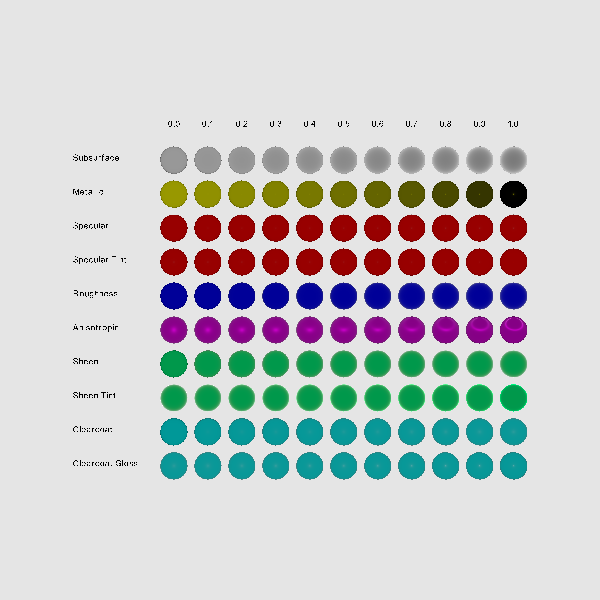# Principled BRDF shader on spheres¶

The Principled Bidirectional Reflectance Distribution Function ([BRDF] (https://en.wikipedia.org/wiki/Bidirectional_reflectance_distribution_function) ) was introduced by Brent Burley as part of the [SIGGRAPH 2012 Physically Based Shading course] (https://blog.selfshadow.com/publications/s2012-shading-course/). Although it is not strictly physically based, it was designed so the parameters included could model materials in the [MERL 100](https://www.merl.com/brdf/) (Material Exchange and Research Library) database. Moreover, each parameter was carefully chosen and limited to be easy to use and understand, so that blending multiple layers together would give intuitive results.

In this demo, we showcase our implementation of the Principled BRDF in FURY.

Let’s start by importing the necessary modules:

```from fury import actor, material, window
import numpy as np
```

Now set up a new scene.

```scene = window.Scene()
scene.background((.9, .9, .9))
```

Let’s define the parameters needed for our demo. In this demo we will see the effect of each one of the 10 parameters defined by the Principled shader. For interpretability and usability purposes, each parameter is limited to values between the range 0 to 1.

```material_params = [
[(1, 1, 1), {'subsurface': 0, 'subsurface_color': [.8, .8, .8]}],
[[1, 1, 0], {'metallic': 0}], [(1, 0, 0), {'specular': 0}],
[(1, 0, 0), {'specular_tint': 0, 'specular': 1}],
[(0, 0, 1), {'roughness': 0}],
[(1, 0, 1), {'anisotropic': 0, 'metallic': .25, 'roughness': .5}],
[[0, 1, .5], {'sheen': 0}], [(0, 1, .5), {'sheen_tint': 0, 'sheen': 1}],
[(0, 1, 1), {'clearcoat': 0}],
[(0, 1, 1), {'clearcoat_gloss': 0, 'clearcoat': 1}]
]
```

We can start to add our actors to the scene and see how different values of the parameters produce interesting effects.

```for i in range(10):
center = [[0, -5 * i, 0]]
for j in range(11):
center = -25 + 5 * j
theta=32, phi=32)
keys = list(material_params[i])
material_params[i][keys] = np.round(0.1 * j, decimals=1)
material.manifest_principled(sphere, **material_params[i])
```

Finally, let’s add some labels to guide us through our visualization.

```labels = ['Subsurface', 'Metallic', 'Specular', 'Specular Tint', 'Roughness',
'Anisotropic', 'Sheen', 'Sheen Tint', 'Clearcoat', 'Clearcoat Gloss']

for i in range(10):
pos = [-40, -5 * i, 0]
label = actor.label(labels[i], pos=pos, scale=(.8, .8, .8),
color=(0, 0, 0))

for j in range(11):
pos = [-26 + 5 * j, 5, 0]
label = actor.label(str(np.round(j * 0.1, decimals=1)), pos=pos,
scale=(.8, .8, .8), color=(0, 0, 0))
```

Out:

```/Users/koudoro/Software/fury/docs/tutorials/03_shaders/viz_principled_spheres.py:72: DeprecationWarning: Label function has been renamed vector_text

* deprecated from version: 0.7.1
* Will raise <class 'fury.deprecator.ExpiredDeprecationError'> as of version: 0.9.0
color=(0, 0, 0))
/Users/koudoro/Software/fury/docs/tutorials/03_shaders/viz_principled_spheres.py:78: DeprecationWarning: Label function has been renamed vector_text

* deprecated from version: 0.7.1
* Will raise <class 'fury.deprecator.ExpiredDeprecationError'> as of version: 0.9.0
scale=(.8, .8, .8), color=(0, 0, 0))
```

And visualize our demo.

```interactive = False
if interactive:
window.show(scene)

window.record(scene, size=(600, 600), out_path="viz_principled_spheres.png")
```Total running time of the script: ( 0 minutes 2.363 seconds)

Gallery generated by Sphinx-Gallery